# Kernels¶

Definition of a hierarchy of classes for kernel functions to be used in convolution, e.g., for data smoothing (low pass filtering) or firing rate estimation.

Examples of usage:
>>> kernel1 = kernels.GaussianKernel(sigma=100*ms)
>>> kernel2 = kernels.ExponentialKernel(sigma=8*mm, invert=True)

class elephant.kernels.AlphaKernel(sigma, invert=False)[source]

Class for alpha kernels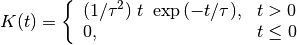with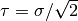.

For the alpha kernel an analytical expression for the boundary of the integral as a function of the area under the alpha kernel function cannot be given. Hence in this case the value of the boundary is determined by kernel-approximating numerical integration, inherited from the Kernel class.

Derived from:

This is the base class for commonly used kernels.

General definition of kernel: A function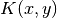is called a kernel function if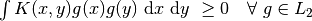Currently implemented kernels are:
• rectangular
• triangular
• epanechnikovlike
• gaussian
• laplacian
• exponential (asymmetric)
• alpha function (asymmetric)

In neuroscience a popular application of kernels is in performing smoothing operations via convolution. In this case, the kernel has the properties of a probability density, i.e., it is positive and normalized to one. Popular choices are the rectangular or Gaussian kernels.

Exponential and alpha kernels may also be used to represent the postynaptic current / potentials in a linear (current-based) model.

Parameters: sigma : Quantity scalar Standard deviation of the kernel. invert: bool, optional If true, asymmetric kernels (e.g., exponential or alpha kernels) are inverted along the time axis. Default: False min_cutoff

Methods

 __call__(t) Evaluates the kernel at all points in the array t. boundary_enclosing_area_fraction(fraction) Calculates the boundary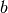so that the integral from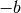toencloses a certain fraction of the integral over the complete kernel. is_symmetric() In the case of symmetric kernels, this method is overwritten in the class SymmetricKernel, where it returns ‘True’, hence leaving the here returned value ‘False’ for the asymmetric kernels. median_index(t) Estimates the index of the Median of the kernel.
class elephant.kernels.EpanechnikovLikeKernel(sigma, invert=False)[source]

Class for epanechnikov-like kernels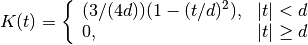with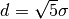being the half width of the kernel.

The Epanechnikov kernel under full consideration of its axioms has a half width of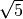. Ignoring one axiom also the respective kernel with half width = 1 can be called Epanechnikov kernel. ( https://de.wikipedia.org/wiki/Epanechnikov-Kern ) However, arbitrary width of this type of kernel is here preferred to be called ‘Epanechnikov-like’ kernel.

Besides the standard deviation sigma, for consistency of interfaces the parameter invert needed for asymmetric kernels also exists without having any effect in the case of symmetric kernels.

Derived from:

Base class for symmetric kernels.

Derived from:

This is the base class for commonly used kernels.

General definition of kernel: A functionis called a kernel function ifCurrently implemented kernels are:
• rectangular
• triangular
• epanechnikovlike
• gaussian
• laplacian
• exponential (asymmetric)
• alpha function (asymmetric)

In neuroscience a popular application of kernels is in performing smoothing operations via convolution. In this case, the kernel has the properties of a probability density, i.e., it is positive and normalized to one. Popular choices are the rectangular or Gaussian kernels.

Exponential and alpha kernels may also be used to represent the postynaptic current / potentials in a linear (current-based) model.

Parameters: sigma : Quantity scalar Standard deviation of the kernel. invert: bool, optional If true, asymmetric kernels (e.g., exponential or alpha kernels) are inverted along the time axis. Default: False min_cutoff

Methods

 __call__(t) Evaluates the kernel at all points in the array t. boundary_enclosing_area_fraction(fraction) Calculates the boundaryso that the integral fromtoencloses a certain fraction of the integral over the complete kernel. is_symmetric() In the case of symmetric kernels, this method is overwritten in the class SymmetricKernel, where it returns ‘True’, hence leaving the here returned value ‘False’ for the asymmetric kernels. median_index(t) Estimates the index of the Median of the kernel.
boundary_enclosing_area_fraction(fraction)[source]

Calculates the boundaryso that the integral fromtoencloses a certain fraction of the integral over the complete kernel. By definition the returned value of the method boundary_enclosing_area_fraction is hence non-negative, even if the whole probability mass of the kernel is concentrated over negative support for inverted kernels.

Returns: Quantity scalar Boundary of the kernel containing area fraction under the kernel density. For Epanechnikov-like kernels, integration of its density within the boundaries 0 and :math:b, and then solving for :math:b leads to the problem of finding the roots of a polynomial of third order. The implemented formulas are based on the solution of this problem given in https://en.wikipedia.org/wiki/Cubic_function, where the following 3 solutions are given: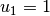: Solution on negative side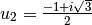: Solution for larger values than zero crossing of the density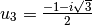: Solution for smaller values than zero crossing of the density The solution :math:u_3 is the relevant one for the problem at hand, since it involves only positive area contributions.
class elephant.kernels.ExponentialKernel(sigma, invert=False)[source]

Class for exponential kernels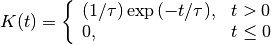with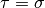.

Derived from:

This is the base class for commonly used kernels.

General definition of kernel: A functionis called a kernel function ifCurrently implemented kernels are:
• rectangular
• triangular
• epanechnikovlike
• gaussian
• laplacian
• exponential (asymmetric)
• alpha function (asymmetric)

In neuroscience a popular application of kernels is in performing smoothing operations via convolution. In this case, the kernel has the properties of a probability density, i.e., it is positive and normalized to one. Popular choices are the rectangular or Gaussian kernels.

Exponential and alpha kernels may also be used to represent the postynaptic current / potentials in a linear (current-based) model.

Parameters: sigma : Quantity scalar Standard deviation of the kernel. invert: bool, optional If true, asymmetric kernels (e.g., exponential or alpha kernels) are inverted along the time axis. Default: False min_cutoff

Methods

 __call__(t) Evaluates the kernel at all points in the array t. boundary_enclosing_area_fraction(fraction) Calculates the boundaryso that the integral fromtoencloses a certain fraction of the integral over the complete kernel. is_symmetric() In the case of symmetric kernels, this method is overwritten in the class SymmetricKernel, where it returns ‘True’, hence leaving the here returned value ‘False’ for the asymmetric kernels. median_index(t) Estimates the index of the Median of the kernel.
boundary_enclosing_area_fraction(fraction)[source]

Calculates the boundaryso that the integral fromtoencloses a certain fraction of the integral over the complete kernel. By definition the returned value of the method boundary_enclosing_area_fraction is hence non-negative, even if the whole probability mass of the kernel is concentrated over negative support for inverted kernels.

Returns: Quantity scalar Boundary of the kernel containing area fraction under the kernel density.
class elephant.kernels.GaussianKernel(sigma, invert=False)[source]

Class for gaussian kernels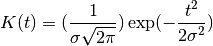with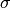being the standard deviation.

Besides the standard deviation sigma, for consistency of interfaces the parameter invert needed for asymmetric kernels also exists without having any effect in the case of symmetric kernels.

Derived from:

Base class for symmetric kernels.

Derived from:

This is the base class for commonly used kernels.

General definition of kernel: A functionis called a kernel function ifCurrently implemented kernels are:
• rectangular
• triangular
• epanechnikovlike
• gaussian
• laplacian
• exponential (asymmetric)
• alpha function (asymmetric)

In neuroscience a popular application of kernels is in performing smoothing operations via convolution. In this case, the kernel has the properties of a probability density, i.e., it is positive and normalized to one. Popular choices are the rectangular or Gaussian kernels.

Exponential and alpha kernels may also be used to represent the postynaptic current / potentials in a linear (current-based) model.

Parameters: sigma : Quantity scalar Standard deviation of the kernel. invert: bool, optional If true, asymmetric kernels (e.g., exponential or alpha kernels) are inverted along the time axis. Default: False min_cutoff

Methods

 __call__(t) Evaluates the kernel at all points in the array t. boundary_enclosing_area_fraction(fraction) Calculates the boundaryso that the integral fromtoencloses a certain fraction of the integral over the complete kernel. is_symmetric() In the case of symmetric kernels, this method is overwritten in the class SymmetricKernel, where it returns ‘True’, hence leaving the here returned value ‘False’ for the asymmetric kernels. median_index(t) Estimates the index of the Median of the kernel.
boundary_enclosing_area_fraction(fraction)[source]

Calculates the boundaryso that the integral fromtoencloses a certain fraction of the integral over the complete kernel. By definition the returned value of the method boundary_enclosing_area_fraction is hence non-negative, even if the whole probability mass of the kernel is concentrated over negative support for inverted kernels.

Returns: Quantity scalar Boundary of the kernel containing area fraction under the kernel density.
class elephant.kernels.Kernel(sigma, invert=False)[source]

This is the base class for commonly used kernels.

General definition of kernel: A functionis called a kernel function ifCurrently implemented kernels are:
• rectangular
• triangular
• epanechnikovlike
• gaussian
• laplacian
• exponential (asymmetric)
• alpha function (asymmetric)

In neuroscience a popular application of kernels is in performing smoothing operations via convolution. In this case, the kernel has the properties of a probability density, i.e., it is positive and normalized to one. Popular choices are the rectangular or Gaussian kernels.

Exponential and alpha kernels may also be used to represent the postynaptic current / potentials in a linear (current-based) model.

Parameters: sigma : Quantity scalar Standard deviation of the kernel. invert: bool, optional If true, asymmetric kernels (e.g., exponential or alpha kernels) are inverted along the time axis. Default: False

Methods

 __call__(t) Evaluates the kernel at all points in the array t. boundary_enclosing_area_fraction(fraction) Calculates the boundaryso that the integral fromtoencloses a certain fraction of the integral over the complete kernel. is_symmetric() In the case of symmetric kernels, this method is overwritten in the class SymmetricKernel, where it returns ‘True’, hence leaving the here returned value ‘False’ for the asymmetric kernels. median_index(t) Estimates the index of the Median of the kernel.
boundary_enclosing_area_fraction(fraction)[source]

Calculates the boundaryso that the integral fromtoencloses a certain fraction of the integral over the complete kernel. By definition the returned value of the method boundary_enclosing_area_fraction is hence non-negative, even if the whole probability mass of the kernel is concentrated over negative support for inverted kernels.

Returns: Quantity scalar Boundary of the kernel containing area fraction under the kernel density.
is_symmetric()[source]

In the case of symmetric kernels, this method is overwritten in the class SymmetricKernel, where it returns ‘True’, hence leaving the here returned value ‘False’ for the asymmetric kernels.

median_index(t)[source]

Estimates the index of the Median of the kernel. This parameter is not mandatory for symmetrical kernels but it is required when asymmetrical kernels have to be aligned at their median.

Returns: int Index of the estimated value of the kernel median.
class elephant.kernels.LaplacianKernel(sigma, invert=False)[source]

Class for laplacian kernels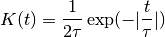with.

Besides the standard deviation sigma, for consistency of interfaces the parameter invert needed for asymmetric kernels also exists without having any effect in the case of symmetric kernels.

Derived from:

Base class for symmetric kernels.

Derived from:

This is the base class for commonly used kernels.

General definition of kernel: A functionis called a kernel function ifCurrently implemented kernels are:
• rectangular
• triangular
• epanechnikovlike
• gaussian
• laplacian
• exponential (asymmetric)
• alpha function (asymmetric)

In neuroscience a popular application of kernels is in performing smoothing operations via convolution. In this case, the kernel has the properties of a probability density, i.e., it is positive and normalized to one. Popular choices are the rectangular or Gaussian kernels.

Exponential and alpha kernels may also be used to represent the postynaptic current / potentials in a linear (current-based) model.

Parameters: sigma : Quantity scalar Standard deviation of the kernel. invert: bool, optional If true, asymmetric kernels (e.g., exponential or alpha kernels) are inverted along the time axis. Default: False min_cutoff

Methods

 __call__(t) Evaluates the kernel at all points in the array t. boundary_enclosing_area_fraction(fraction) Calculates the boundaryso that the integral fromtoencloses a certain fraction of the integral over the complete kernel. is_symmetric() In the case of symmetric kernels, this method is overwritten in the class SymmetricKernel, where it returns ‘True’, hence leaving the here returned value ‘False’ for the asymmetric kernels. median_index(t) Estimates the index of the Median of the kernel.
boundary_enclosing_area_fraction(fraction)[source]

Calculates the boundaryso that the integral fromtoencloses a certain fraction of the integral over the complete kernel. By definition the returned value of the method boundary_enclosing_area_fraction is hence non-negative, even if the whole probability mass of the kernel is concentrated over negative support for inverted kernels.

Returns: Quantity scalar Boundary of the kernel containing area fraction under the kernel density.
class elephant.kernels.RectangularKernel(sigma, invert=False)[source]

Class for rectangular kernels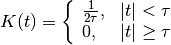with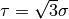corresponding to the half width of the kernel.

Besides the standard deviation sigma, for consistency of interfaces the parameter invert needed for asymmetric kernels also exists without having any effect in the case of symmetric kernels.

Derived from:

Base class for symmetric kernels.

Derived from:

This is the base class for commonly used kernels.

General definition of kernel: A functionis called a kernel function ifCurrently implemented kernels are:
• rectangular
• triangular
• epanechnikovlike
• gaussian
• laplacian
• exponential (asymmetric)
• alpha function (asymmetric)

In neuroscience a popular application of kernels is in performing smoothing operations via convolution. In this case, the kernel has the properties of a probability density, i.e., it is positive and normalized to one. Popular choices are the rectangular or Gaussian kernels.

Exponential and alpha kernels may also be used to represent the postynaptic current / potentials in a linear (current-based) model.

Parameters: sigma : Quantity scalar Standard deviation of the kernel. invert: bool, optional If true, asymmetric kernels (e.g., exponential or alpha kernels) are inverted along the time axis. Default: False min_cutoff

Methods

 __call__(t) Evaluates the kernel at all points in the array t. boundary_enclosing_area_fraction(fraction) Calculates the boundaryso that the integral fromtoencloses a certain fraction of the integral over the complete kernel. is_symmetric() In the case of symmetric kernels, this method is overwritten in the class SymmetricKernel, where it returns ‘True’, hence leaving the here returned value ‘False’ for the asymmetric kernels. median_index(t) Estimates the index of the Median of the kernel.
boundary_enclosing_area_fraction(fraction)[source]

Calculates the boundaryso that the integral fromtoencloses a certain fraction of the integral over the complete kernel. By definition the returned value of the method boundary_enclosing_area_fraction is hence non-negative, even if the whole probability mass of the kernel is concentrated over negative support for inverted kernels.

Returns: Quantity scalar Boundary of the kernel containing area fraction under the kernel density.
class elephant.kernels.SymmetricKernel(sigma, invert=False)[source]

Base class for symmetric kernels.

Derived from:

This is the base class for commonly used kernels.

General definition of kernel: A functionis called a kernel function ifCurrently implemented kernels are:
• rectangular
• triangular
• epanechnikovlike
• gaussian
• laplacian
• exponential (asymmetric)
• alpha function (asymmetric)

In neuroscience a popular application of kernels is in performing smoothing operations via convolution. In this case, the kernel has the properties of a probability density, i.e., it is positive and normalized to one. Popular choices are the rectangular or Gaussian kernels.

Exponential and alpha kernels may also be used to represent the postynaptic current / potentials in a linear (current-based) model.

Parameters: sigma : Quantity scalar Standard deviation of the kernel. invert: bool, optional If true, asymmetric kernels (e.g., exponential or alpha kernels) are inverted along the time axis. Default: False

Methods

 __call__(t) Evaluates the kernel at all points in the array t. boundary_enclosing_area_fraction(fraction) Calculates the boundaryso that the integral fromtoencloses a certain fraction of the integral over the complete kernel. is_symmetric() In the case of symmetric kernels, this method is overwritten in the class SymmetricKernel, where it returns ‘True’, hence leaving the here returned value ‘False’ for the asymmetric kernels. median_index(t) Estimates the index of the Median of the kernel.
is_symmetric()[source]

In the case of symmetric kernels, this method is overwritten in the class SymmetricKernel, where it returns ‘True’, hence leaving the here returned value ‘False’ for the asymmetric kernels.

class elephant.kernels.TriangularKernel(sigma, invert=False)[source]

Class for triangular kernels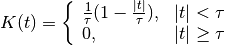with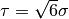corresponding to the half width of the kernel.

Besides the standard deviation sigma, for consistency of interfaces the parameter invert needed for asymmetric kernels also exists without having any effect in the case of symmetric kernels.

Derived from:

Base class for symmetric kernels.

Derived from:

This is the base class for commonly used kernels.

General definition of kernel: A functionis called a kernel function ifCurrently implemented kernels are:
• rectangular
• triangular
• epanechnikovlike
• gaussian
• laplacian
• exponential (asymmetric)
• alpha function (asymmetric)

In neuroscience a popular application of kernels is in performing smoothing operations via convolution. In this case, the kernel has the properties of a probability density, i.e., it is positive and normalized to one. Popular choices are the rectangular or Gaussian kernels.

Exponential and alpha kernels may also be used to represent the postynaptic current / potentials in a linear (current-based) model.

Parameters: sigma : Quantity scalar Standard deviation of the kernel. invert: bool, optional If true, asymmetric kernels (e.g., exponential or alpha kernels) are inverted along the time axis. Default: False min_cutoff

Methods

 __call__(t) Evaluates the kernel at all points in the array t. boundary_enclosing_area_fraction(fraction) Calculates the boundaryso that the integral fromtoencloses a certain fraction of the integral over the complete kernel. is_symmetric() In the case of symmetric kernels, this method is overwritten in the class SymmetricKernel, where it returns ‘True’, hence leaving the here returned value ‘False’ for the asymmetric kernels. median_index(t) Estimates the index of the Median of the kernel.
boundary_enclosing_area_fraction(fraction)[source]

Calculates the boundaryso that the integral fromtoencloses a certain fraction of the integral over the complete kernel. By definition the returned value of the method boundary_enclosing_area_fraction is hence non-negative, even if the whole probability mass of the kernel is concentrated over negative support for inverted kernels.

Returns: Quantity scalar Boundary of the kernel containing area fraction under the kernel density.
elephant.kernels.inherit_docstring(fromfunc, sep='')[source]

Decorator: Copy the docstring of fromfunc

based on: http://stackoverflow.com/questions/13741998/ is-there-a-way-to-let-classes-inherit-the-documentation-of-their-superclass-with